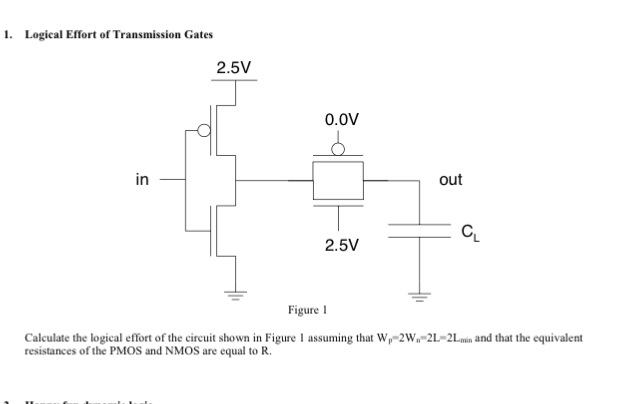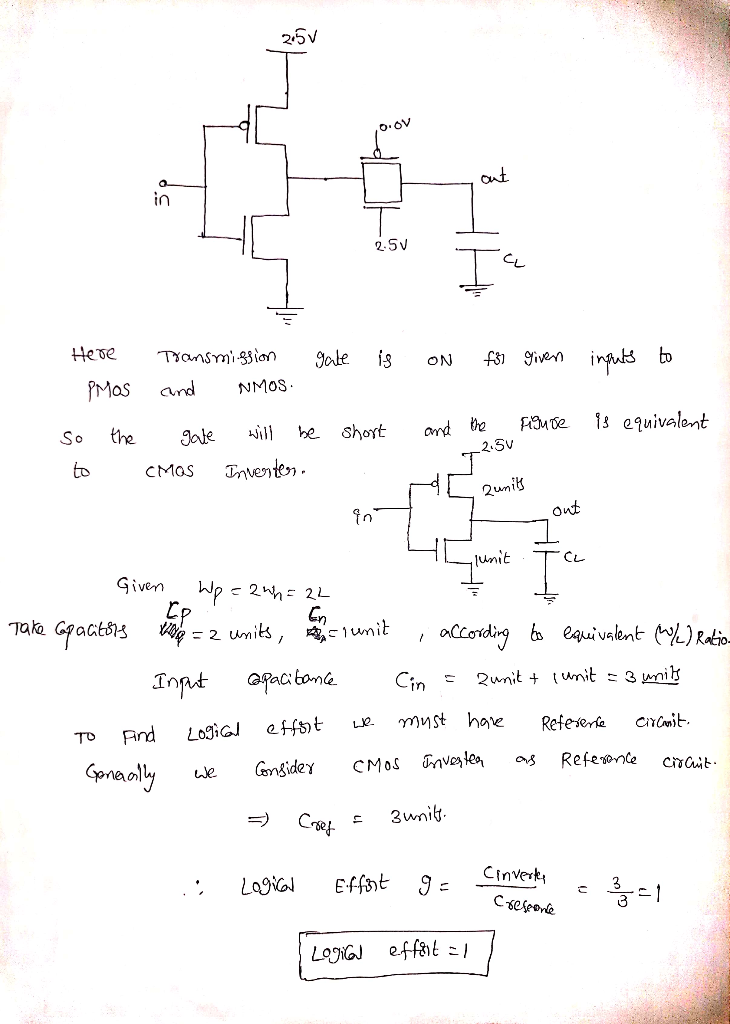# 1. Logical Effort of Transmission Gates 2.5V 0.0V in out 2.5V Figure 1 Calculate the logical effo...1. Logical Effort of Transmission Gates 2.5V 0.0V in out 2.5V Figure 1 Calculate the logical effort of the circuit shown in Figure 1 assuming that W-W2-2min and that the equivalent resistances of the PMOS and NMOS are equal to R##### Add Answer of: 1. Logical Effort of Transmission Gates 2.5V 0.0V in out 2.5V Figure 1 Calculate the logical effo...
More Homework Help Questions Additional questions in this topic.